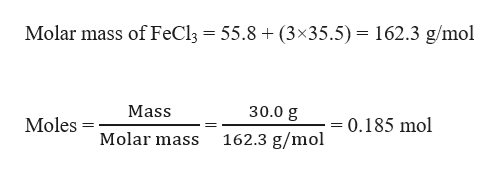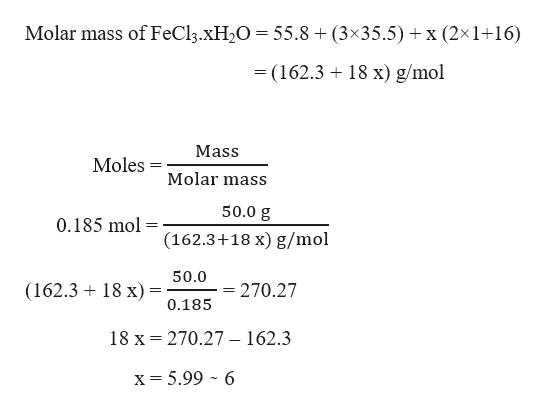A 50.0 mg sample of FeCl3·xH2O was heated to drive off the waters of hydration. After cooling to room temperature, the resulting residue weighed 30.0 mg. Determine the chemical formula of the hydrate, and name the hydrate.

Question

A 50.0 mg sample of FeCl3·xH2O was heated to drive off the waters of hydration. After cooling to room temperature, the resulting residue weighed 30.0 mg. Determine the chemical formula of the hydrate, and name the hydrate.

Step 1

Given,

Mass of sample of FeCl3.xH2O = 50.0 g

After driving off water from sample, mass of FeCl3 = 30.0 g

Moles of FeCl3 can be calculated as:help_outlineImage TranscriptioncloseMolar mass of FeCl3 55.8 (3x35.5) = 162.3 g/mol Mass 30.0 g Moles 0.185 mol Molar mass 162.3 g/mol fullscreen
Step 2

It is clear that, 1 mole of FeCl3.xH2O contains 1 mole of FeCl3.

So, moles of FeCl3.xH2O = moles of FeCl3 = 0.185 mol

Therefore...help_outlineImage TranscriptioncloseMolar mass of FeCl3.XH2O = 55.8 + (3x35.5) x (2x1+16) - (162.3 18 x) g/mol Mass Moles Molar mass 50.0 g 0.185 mol (162.3+18 x) g/mol 50.0 (162.3 18 x)= = 270.27 0.185 18 x 270.27 - 162.3 x= 5.99 6 fullscreen

Want to see the full answer?

See Solution

Want to see this answer and more?

Our solutions are written by experts, many with advanced degrees, and available 24/7

See Solution
Tagged in

General Chemistry